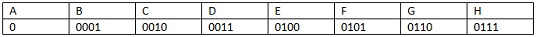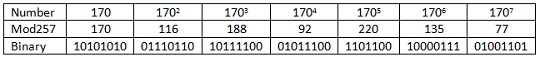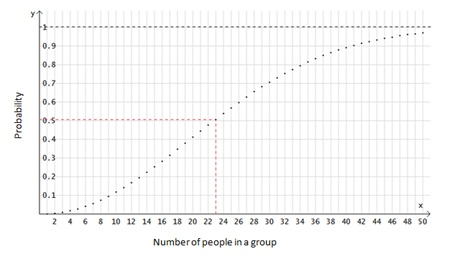# The Weakest Link in Many Cryptosystems – Part 1 of 2

by Peter Landrock on 13. November 2012

### Introduction

It is well-known and appreciated by most users - even if often ignored(!) - that if you choose a weak password, you are exposing yourself to various risks. Whether your password is used for encryption of confidential data or just for access control doesn't really matter, so let's assume for a minute that it is actually used to encrypt your data - or perhaps to encrypt a key that is used to encrypt your data. The situation you are in is that you are using a random bit generator of poor quality (in this case yourself!) to generate your key, in the sense that the output - your password - can easily be guessed or predicted.

One aspect to keep in mind here is the size of the space in which your password theoretically might be chosen, which is determined from the length (in bits or number of digits or whatever). A PIN-code for a debit- or credit card is 4 digits, which yields 104 = 10,000 possibilities. The number is limited to 4, as you need to be able to memorise it, and 4 digits is considered the limit for most humans. This very small number is then compensated by the fact that you will only have three attempts to key in the right PIN at an ATM or POS-terminal. In contrast, for encryption schemes, the key must be so long that it is not feasible to run through all the combinations in a methodical manner within a reasonable timescale, also known as exhaustive search.

Most of us constantly use cryptosystems, whether transparent to us such as in an underlying SSL protocol, or by conscious choice, such as use of S/MIME or PGP or whatever. In most scenarios, you do not have to choose the key to be used, in fact most of the time you cannot even influence the generation of it. So how do you know if it has been properly generated, and what exactly does that entail? Well you don't, that's the problem.

The main point of this article is that it is not enough to have a strong algorithm with a sufficiently long key. There is at least one more important aspect - apart from secure storage and protection of course. The missing link is known as randomness, or entropy.### Randomness

Mathematically speaking, we have a means for measuring randomness. Not in one particular key, or one bit string, but in a variable such as a distribution of keys. This measure is known as the entropy, or Shannon entropy, which was introduced in a famous paper by Claude Shannon in 1948 [S]. Interestingly enough, this theory has a lot in common with the theory of entropy in thermodynamics - but that is another story. Suffices it here to mention that the higher the entropy of a system being processed on a processor, the higher the heat radiation from the processor.

Going back to Shannon entropy, it measures how random a variable is, i.e. what can be predicted about the expected values. If the variable is to flip a coin, there is 1 bit of information in the outcome, and if you spin the coin 3 times, there are 3 bits of information in the outcome. In contrast, if the variable is not evenly distributed, then even if there still are 8 options, the entropy may be less than 3 bits. One aspect of Shannon's theory is that this implies you need less than 3 bits on average to describe the value of the variable. For instance if A occurs with probability 93%, and B,C,D,E,FG and H each occur with probability 1% you can use the coding in the diagram below (known as Huffman coding), and you will discover that the average number of bits you need to describe an event is 1.21 rather than 3. So the smaller the entropy, the less therandomness of the variable. In the extreme, an entropy of 0 means that the outcome is always the same. For more on information entropy, we refer to the first book on the subject, [SW], which has been reprinted several times, or [MOV].

### Seeds and Pseudo RandomnessGiven a key, there is normally no way you can decide if it is chosen sufficiently random or not. As an example, consider all binary keys of length 8. Most people would feel that 10101010 does not look random at all, whereas keys like 01110110, 10111100, 01011100, 10000111 and 01001101 perhaps do? Yet they are all connected, and in a very simple manner. Indeed, just as 376 is interpreted as 3x102+7x101+6x1, the binary number 10101010 is the number 1x27+1x25+1x23+1x2 = 128+32+8+2 = 170. Now take the (prime) 257 (= 100000001 in binary) and calculate 1702 mod 257 by which we mean the remainder you get when you divide 1702 with 257, which is 116 as 1702 = 28900 and 28900 = 112x257 + 116. Now write 116 as a binary number, which is done in the following way: Subtract the largest possible power of 2, which is 64 = 26, and continue in the fashion: 116 = 64 + 32 + 16 + 4 +2, or as a binary number of length 8: 01110110. Continue with 1703 mod 257 = 116x170 mod 257 = 188, or in binary form, 10111100. Moving on to 1704, we get 92 or, 01011100, then 1705 which yields 220 or 11011100, etc.

.You will recognize the sequence thus generated as the sequence we listed above. It may look random, yet it is not random at all as we have just shown. Nevertheless if we scale this approach and start with a random source, we have a good way of mass producing what is known as pseudorandom numbers. More on this later.

Most people have a good feeling for achieving randomness: flip a coin, or spin a racket, and let another person choose preferably as soon as you have starting flipping or spinning, but before the result becomes predictable. This works well in principle, but is by far too tedious for cryptographic keys. Triple DES requires 112 bits, and AES typically even more.

However, if we can get hold of one truly random bit sequence of sufficient length - called a seed - we can use the idea sketched above to make as many - not random, but pseudo-random - bits as we need. By pseudo-random we simply mean that statistically, they behave as if they really were truly random. This is not entirely true actually, we need one thing more: an algorithm, or method, that will generate bits and has the property that if you can predict the next bit with a probability larger than ½, you can solve a hard mathematical problem that mathematicians have not be able to solve so far, such as factoring a composite number into prime numbers. That is precisely what we demonstrated above using the prime 257.  If instead of 257 we use a product of two (large) primes, we actually know (meaning that we can give a mathematical proof) that given a product of two primes, say n, if we can predict the lowest bit of m2 mod n for various m, then we can factor n. This is known as the Blum-Blum-Shub pseudorandom bit generator. For more we refer to [MOV].

Most professional cryptographic libraries have proper algorithms for generating pseudo-random numbers, so the Achilles heel is the initial random seed. This cannot be provided by the library, as it is de facto a secret key in most solutions. And this is the culprit in most scenarios, where the solution is realised with weak keys. Indeed, the software engineer who integrates the crypto library into some larger solution often lacks skills to find a sufficiently strong source for the initial seed. This is the course of the problem. Suppose - just for the sake of the argument - that he takes the time and the date. Even if this is down to one hundredth of a second, the total space of choice is 24x3600x100 or about 8.6 mill, which is only about 23 bits of information and thus is prone to an exhaustive search attack.

In a school class of unrelated pupils (that is, no twins!) and assuming birthdays are evenly distributed, how many pupils do you need on average in the class in order for the probability that at least 2 have a birthday in common is ½?

Well, surprisingly, it turns out to be 23 only!

This is not that hard to calculate: Assume there are n children in the class, and let's calculate the probability that none have their birthday on the same day. The total number of combinations is 365n. The number of combinations where none have their birthday on the same day is calculated as follows: If there is only one, it is obviously 365. If there are several pupils, the second will only have 364 options, the third 363 options, and so on. So the probability of no common birthday is 365x364x363x....x(365-n)/365n. Obviously, as n increases, this number gets smaller and smaller, and the first time it moves below ½ turns out to be already when n = 23.This has the following implication for cryptographic functions and in particular attacks on these: Consider a function f that maps bit sequences to bit sequences of a fixed length n. So the maximal number of images under this function is 2n. What we are after is the situation where two different sequences, x and y, are mapped to the same image, i.e. f(x) = f(y), known as a collision. The function f could be a hash function, or it could be - - a key generation programme!

The calculation given above can be generalised, and it turns out that for large image spaces (e.g. n large), and a random function f, we can expect collisions with probability ½ if we calculate about 1.25∙2n/2 (and in the example above comes out at 1.25∙√365 = 23.88) values. This means that in the scenario above, if people would - sometimes and perhaps too often even - take the caution and use a random timestamp down to 1/100th of a second, we would amongst all keys generated only need to choose about 1.25√8.6mil = 3665 keys to find a collision with probability ½. A collision here would mean that people were using the same secret key because of a lousy key generation procedure.

### References

[MOV] Menezes, Alfred J., van Oorschot, Paul C. & Vanstone, Scott A., Handbook of Applied Cryptography, CRC Press, 1997.

[S] Shannon, Claude E. (July/October 1948). "A Mathematical Theory of Communication". Bell System Technical Journal 27 (3): 379-423.

[SW] Shannon, Claude E. & Weaver, Warren. The Mathematical Theory of Communication. Univ of Illinois Press, 1949.

## Want to know how we can help ?

Get in touch to better understand how our solutions secure ecommerce and billions of transactions worldwide.